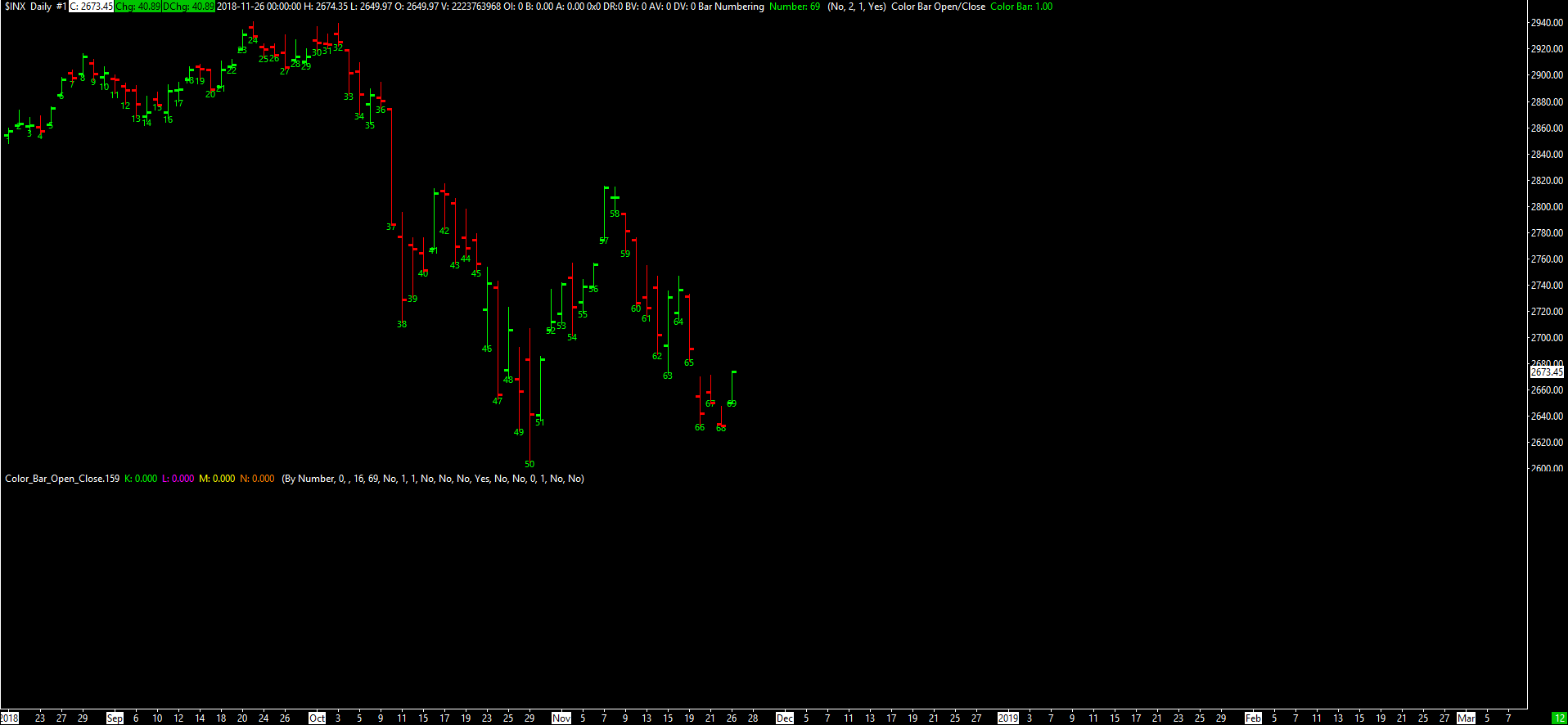# Technical Studies Reference

### Color Bar Open/Close

This study colors the chart bars based on whether the bar's Open Price is higher or lower than its Close Price.

Let $$O_t$$ and $$C_t$$ be the values of the Open and Close Prices, respectively, at Index $$t$$. This study colors the chart bar at Index $$t$$ according to the following rules.

• If $$C_t > O_t$$, then the entire main price graph bar is colored with the Primary Color of the study (the first color button of the Color Bar Subgraph).
• If $$C_t < O_t$$, then the entire main price graph bar is colored with the Secondary Color of the study (the second color button of the Color Bar Subgraph).
• If $$C_t = O_t$$ and $$C_{t - 1} \geq O_{t - 1}$$, then the entire main price graph bar is colored with the Primary Color of the study.
• If $$C_t = O_t$$ and $$C_{t - 1} < O_{t - 1}$$, then the entire main price graph bar is colored with the Secondary Color of the study.

For this study to work correctly, the Color Bar Subgraph Draw Style must be set to Color Bar.

The output value is $$1$$ for all $$t$$.

#### Inputs

This study has no Inputs.